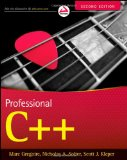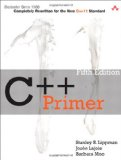# C++11 lambda tutorial

## Posted on November 1, 2011 by Paul

Don’t forget to check my short introduction to generic lambdas for C++14.

In my previous tutorials I’ve presented some of the newest C++11 additions to the language: regular expressions and raw strings. Another useful language addition is lambda, a lambda expression allows you to define and use anonymous functions inline (functions with a body but without a name). You can use a lambda expression instead of a function object, avoiding the need to create a separate class and a function definition. This is useful for example when you need a one-line expression to interact with many of the STL algorithms.

Lambda expressions are currently available in all major C++ compilers GCC, Visual Studio and Clang.

Let’s create a (useless) simple example of lambda expression that will return a number:

Obviously the above code will do nothing visible (actually it will return an integer), we can use it to print 4 on the console:

A slightly more useful example that will receive as input an integer and will return twice this number:

From the above examples the syntax of a lambda expression can be written (this is not the complete syntax of a lambda expression):

A lambda expression that will add two numbers and will return the result can be written as:

We can use a lambda expression inside another lambda expression, combining the above examples we can create a slightly more complex example:

If you need to use more than once a lambda expression it could be cumbersome to copy the same piece of code, you can use a pointer to the lambda expression:

The auto keyword will take care to define func as a pointer to the lambda expression.

Suppose we need to sort a vector of integers, using the sort algorithm from C++98 this can be implemented:

Using a lambda expression we can achieve the same result by avoiding to declare a compare function:

If you are interested in learning more about the new C++11 syntax I would recommend reading Professional C++ by M. Gregoire, N. A. Solter, S. J. Kleper 2nd edition:or, if you are a C++ beginner you could read C++ Primer (5th Edition) by S. B. Lippman, J. Lajoie, B. E. Moo.